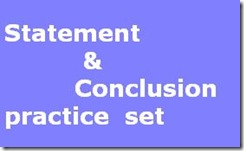## Tuesday, 9 April 2013Directions (1-6) : In each of the questions below are given three statements followed by the three Conclusions numbered, I II and III. You have to take the given statements to be true even if they seem to be at variance from commonly known facts. Read all the Conclusions and then decide which of the given Conclusions logically follows from the given statements disregarding commonly known facts.

1. Statements: Some tents are buildings. Some buildings are chairs. Some chairs are windows.

Conclusions:

I. Some windows are buildings.

II. Some windows are tents.

JÍI. Some chairs are tents.

[A]None follows

[B] Only I and II follow

[C] Only II and III follow

[D] Only I and III follow

[E] All follow

ANS. [A]

2. Statements:

All tables are boards.

All pens are boards.

All boards are papers.

Concussions:

I. Some pens are tables.

IL Some papers are pens.

III. No pen is table.

[A] All follow

[B] Only either I or III follows

[C]' Only II follows

[D] Only either I or III and II follow

[E] None of these

ANS. [D]

3. Statements:

All chains are cycles.

Some cycles are wheels.

All wheels are mirrors.

Conclusions:

I. Some cycles are mirrors.

II. Some wheels are chains.

III. Some mirrors are cycles.

[A] None follows

[B] Only I follows

[C] Only II follows

[D] Only I and III follow

[E] All follow

ANS. [D]

4. Statements:

Some boxes are hammers.

Conclusions:

I. Some rings are hammers.

II. Some hammers are boxes.

III. Some rings are boxes.

[A] None follows

[B] Only I follows

[C] Only II and III follow

[D] Only I and II follow

[E] All follow

ANS. [D]

5. Statements:

Ail jackets are trousers.

No trouser is shirt.

Some shirts are caps.

Conclusions:

I. Some caps are jackets.

II. Some shirts are jackets.

III. Some trousers are jackets.

[A] Only I follows

[B] Only II follows

[C] Only III follows

[D] All follow

[E] None of these

ANS. [C]

6. Statements:

Some cameras are binoculars.

All binoculars are spoons.

Some spoons are cups.

Conclusions:

I. Some cups are binoculars.

II. Some spoons are cameras.

III. Some cups are cameras.

[A] Only I follows

[B] Only II follows

[C] Only III follows

[D] Only either I or II follows

[E] None of these

ANS. [B]

Directions (7-10): Study the following arrangement carefully and answer the questions given below :R D 1 5 E % K 3 J I 9 P 8 # A 2 B\$ K M 6 W @ N 4 © T * 7 F H

7. Which of the following is the third to the right of the twelfth from the left end ?

[A] J

[B]A

[C] B

[D] @

[E] None of these

ANS. [B]

8. How many such numbers are there in the above arrangement, each of which is immediately preceded by a consonant and immediately followed by a symbol?

[A]One

[B] Two

[C] Three

[D] Four

[D] None of these

ANS. [B]

9. Four of the following five are alike in a certain way based on their positions in the above arrangement and so form a group.

Which is the one that does not belong to that group ?

[A] B K 2

[B] E 1 %

[C] J 9 3

[E] 4 T N

ANS. [B]

10. What should come in place of the question mark (?) in the following series based on the above arrangement ?

D 5 % J 9 8 2 \$ M ?

[A] @ 4 T

[E] None of these

ANS. [A]

1.sir

I get 54.80 in ibps com.result of cwe and inter. from obc gujarat
what is my chance to get job in a bank ?

Gautam vara

2.Hello
how to crack the statement and conclusion question specificaly questions with "some,no,all"?

3.Hello ,
How to crack the statement and conclusion question specifically with words "some,no,all"?